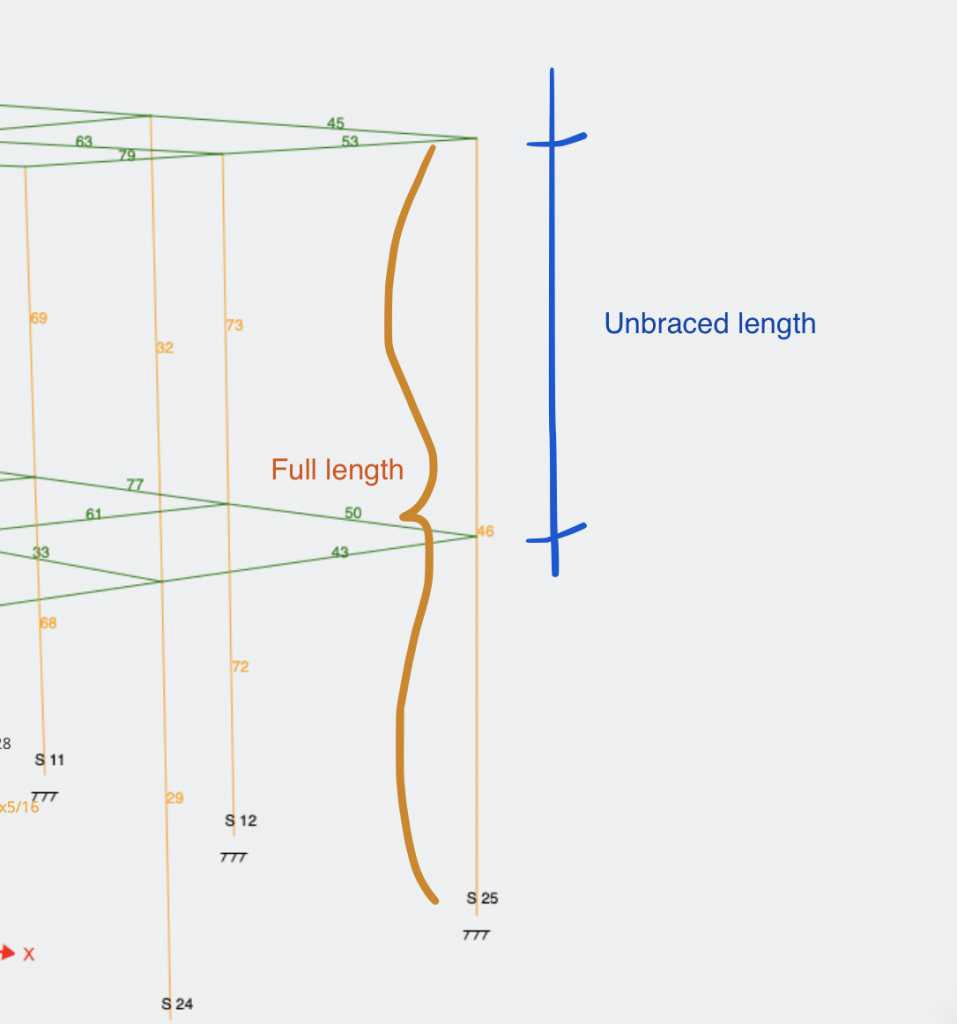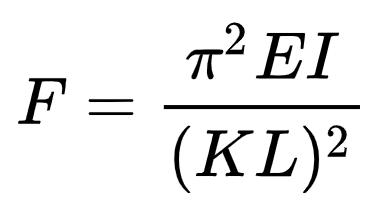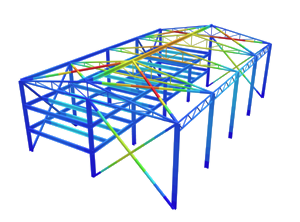SkyCiv Documentation

Your guide to SkyCiv software - tutorials, how-to guides and technical articles

1. Home
2. SkyCiv Member Design
3. General
4. A Guide to Unbraced Lengths, Effective Length Factor (K), and Slenderness

# A Guide to Unbraced Lengths, Effective Length Factor (K), and Slenderness

## A Guide to Effective Lengths, Slenderness and K Determination

SkyCiv’s 3D modeling and analysis tool, Structural 3D, allows users to not only run Linear and Non-Linear Analysis, but it also supports Buckling Analysis, which can sometimes be an afterthought in the structural engineering process. This article will go talk about the parameters that are used to describe a member’s resistance to buckling failure, and how that looks in SkyCiv Structural 3D. While there are other forms of buckling like lateral-torsional buckling, plate buckling, etc., this article will strictly look at buckling in compression members.

Buckling is a form of failure in compression members, which are usually columns. Consider the simple example of a soda can – when the can is compressed from either end, at a given force, there will a sudden deflection and it will collapse in on itself at some point along the length of the can. This is due to the occurrence of buckling. For structural elements, this failure mode needs to be considered, as it can have dire consequences on the structural integrity of the entire structure.

## Slenderness Ratio

Members in which buckling becomes the governing failure mechanism are usually long and slender relative to their cross-section. We use something called the slenderness ratio to describe how “slender” a member is. The slenderness ratio is a quick and fairly simple ratio to calculate the buckling phenomena that occurs in a compression member. It is defined as:

slenderness ratio = KL/r

Where is the effective length factor, l is the unbraced length of the member and r is the radius of gyration. The product KL is known simply as the effective length. The radius of gyration is found as follows:

r = sqrt(Ig/Ag)

Note: As approximations, it’s possible to use r = 0.3h for square and rectangular sections, and r = 0.25h for circular sections.

## Unbraced Length

Unbraced length (unsupported length) is the largest distance along the member between brace points, or points at which the member is braced against deflection in the given direction. So, for a free-standing column, the unbraced length would be the full height/length. In many instances, the unbraced length of a member is less than the full length since there are members or other mechanisms bracing it along with the member.

For example, the below column has a full length of 20ft, but the unbraced length in both principal axis directions is 10ft, since there is a beam bracing it at the midpoint of the column.In the SkyCiv Design modules — Member Design and RC Design — the unbraced length is automatically calculated and populated in the Members table. Engineers can adjust and manipulate these values manually should any custom situations occur or special assumptions be made.

## Effective Length Factor

Now that we know what the unbraced length is for a member, we can figure out what the effective length factor is. In SkyCiv Structural 3D, the effective length of a member is determined during a buckling analysis, where the eigenvalue of each member is calculated in order to determine critical buckling forces.

This basically just means that the solver will find the effective length of a member-based on finite element analysis. However, empirical K values are routinely used in practice and can be inferred from the commonly seen table below.

The effective length (K) factor of a member in compression is dependent on the support conditions at each end. The higher the K factor, the more the support conditions hurt the member’s resistance to buckling, and vice versa. Looking at the table below, we can see the effective length factors of common support situations with columns, or other compressive members:

## Buckling Capacity

Now that we can describe members using the slenderness ratio, how is buckling actually checked? The critical stress at which a member will buckle and in essence, its strength can be described with the Euler formula shown below:Where we see the effective length in the denominator, and the Modulus of Elasticity and Moment of Inertia of the section in the numerator. This tells us that the smaller the effective length of a section, as well as the higher the moment of inertia in the axis of analysis, will result in a higher critical load that would buckle the member.

Because most members aren’t fully symmetrical in all directions, members are usually analyzed in both principal directions of the section. In SkyCiv Structural 3D, the principal directions would be the Y and Z axis of a member, which corresponds to the vertical and horizontal axis of a section, respectively, when looking at it in plane view.

### Do all members need to be checked for buckling?

Buckling is a very unique type of failure, and should not be forgotten or written off, but there are a few stipulations and general practices in the industry that allow engineers to disregard buckling as a failure method, only because that member would otherwise fail before it’s critical buckling stress is reached through a different failure method. These stipulations depend on the modulus of elasticity of the member, and therefore the material.

If a column is considered “long”, then it is susceptible to buckling and it should be checked. Otherwise, columns are considered “short” or “intermediate” in which case, buckling is less of a threat. The classification of members as short, intermediate, or long, is done by using the slenderness ratio that we calculated earlier.

For steel members, a slenderness ratio below 50 can be considered “short”. A slenderness ratio greater than 200 tells us the member is “long”, and buckling from compressive forces should be considered. Members with slenderness ratios between those two values are considered “intermediate”, where engineering judgment should be used.

For concrete members, the “short” and “long” designation cutoff occurs at a slenderness ratio of 10.

For wood members, buckling is more unique, since the material itself is not isotropic (the strength of the material varies). However, in most cases, wood members with a slenderness ratio below 10 can be considered “short”.

Overall, the check is fairly straightforward and quick, so most engineers air on the side of caution. Luckily, in SkyCiv Structural 3D, when users queue a buckling analysis, these checks are done for every single member at a fraction of the time.